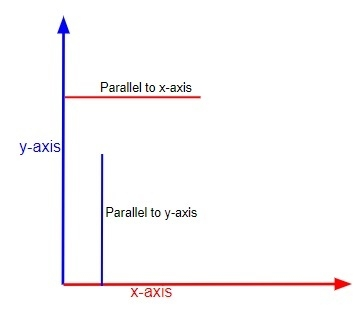# C Program to check if the points are parallel to X axis or Y axis

CServer Side ProgrammingProgramming

Given n number of points we have to check that whether the point is parallel to x-axis or y-axis or no axis according to a graph. A graph is a figure which is used to show a relationship between two variables each measured along with axis at right angles. Parallel are the same lines which are having same distance at all points, like railway tracks are parallel to each other.

So, we have to find whether the points are parallel to x-axis or y-axis means the distance between the coordinates and the axis are same at all points.

## What is an axis

The graph is measured along with two axis’ x-axis and y-axis, both of the axis start from a point value 0 and extend according to their particular variable value. Both of the axis combined form a figure like right angle triangle.

Let’s understand it clearly with help of an simple diagrammatic representation −The approach used below is as follows

• Firstly we take the coordinates of a graph in way of (x, y) coordinates.
• Then check whether they are parallel to which axis.
• If all y coordinates are same, then the graph is parallel to x-axis.
• Else if the x coordinates are same, then the graph is parallel to y-axis.
• Else the graph is not parallel to either of the axis.

## Algorithm

Start
In function void parallel (int n, int a[])
Step 1-> Declare and initialize i and j
Step 2-> Declare bool x = true, y = true
Step 3-> Loop For i = 0 and i < n – 1 and i++
Loop For j = 0 and j < 2 and j++
If a[i] != a[i + 1] then,
Set x as false
If a[i] != a[i + 1] then,
Set y as false
End loop
End loop
Step 4-> If x then,
Print "parallel to X Axis\n"
Step 5-> Else if y
Print "parallel to Y Axis\n"
Step 6-> Else
Print "parallel to X and Y Axis\n"
In function int main()
Step 1-> Declare an array “a[]”
Step 2-> Declare and Initialize n as sizeof(a) / sizeof(a)
Step 3-> Call function parallel(n, a)

## Example

#include <stdio.h>
// To check the line is parellel or not

void parallel(int n, int a[]) {
int i, j;
bool x = true, y = true;
// checking for parallel to X and Y
// axis condition
for (i = 0; i < n - 1; i++) {
for (j = 0; j < 2; j++) {
if (a[i] != a[i + 1])
x = false;
if (a[i] != a[i + 1])
y = false;
}
}
// To display the output
if (x)
printf("parallel to X Axis\n" );
else if (y)
printf("parallel to Y Axis\n" );
else
printf("parallel to X and Y Axis\n" );
}
int main() {
int a[] = { { 2, 1 },
{ 3, 1 },
{ 4, 1 },
{ 0, 1 } };
int n = sizeof(a) / sizeof(a);
parallel(n, a);
return 0;
}

## Output

If run the above code it will generate the following output −

parallel to Y Axis